Courses

# Ex 2.5 NCERT Solutions - Polynomials Class 9 Notes | EduRev

## Mathematics (Maths) Class 9

Created by: Vp Classes

## Class 9 : Ex 2.5 NCERT Solutions - Polynomials Class 9 Notes | EduRev

The document Ex 2.5 NCERT Solutions - Polynomials Class 9 Notes | EduRev is a part of the Class 9 Course Mathematics (Maths) Class 9.
All you need of Class 9 at this link: Class 9

FACTORISATION USING ALGEBRAIC IDENTITIES:

We have used the following identities in our earlier classes:

I . (x + y)2 = x2 + 2xy + y2

II . (x – y)2 = x2 – 2xy + y2

III. x2 – y2 = (x + y)(x – y)

IV. (x + a)(x + b) = x2 + (a + b)x + ab

We shall use these identities to factorise algebraic expressions:

Note: The identity I can be extended to a trinomial such as (x + y + z)2 = (p + z)2

[Using x + y = p] = p2 + 2pz + z2
= (x + y)2 + 2(x + y)z + z2
= x2 + y2 + 2xy + 2xz + 2yz + z2
= x2 + y2 + z2 + 2xy + 2yz + 2zx

∴ Thus, we have: Identity V:  (x + y + z)2
= x+ y2 + z2 + 2xy + 2yz + 2zx

We can also extend the identity–I to complete (x + y)3.

We have: (x + y)= (x + y)(x + y)(x + y)
= (x + y)[(x + y)2]
= (x + y)[x2 + 2xy + y2]
= x[x2 + 2xy + y2] + y[x2 + 2xy + y2]
= [x3 + 2x2y + xy2] + [xy2 + 2xy+ y3]
= x3 + 3x2y + 3xy2 + y3 = x3 + y+ 3xy(x + y)

Thus, we have: Identity VI: (x + y)3= x3 + y3 + 3xy(x + y)

By replacing y by (–y) in identity VI, we have:

Identity VII: (x – y)3 = x3 – y3 – 3xy(x – y)

or (x – y)3 = x3 – 3x2y + 3xy2 – y3

Ques 1. Use suitable identities to find the following products:

(i) (x + 4)(x + 10)

(ii) (x + 8)(x – 10)

(iii) (3x + 4)(3x – 5)

(iv)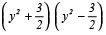(v) (3 – 2x)(3 + 2x)
Solution: (i) (x + 4)(x + 10):
Using the identity (x + a)(x + b)
= x2 + (a + b)x + ab,
we have: (x + 4)(x + 10)
= x2 + (4 + 10)x + (4 x 10)
= x2 + 14x + 40

(ii) (x + 8)(x – 10): Here, a = 8 and b = (–10)
∴ Using (x + a)(x + b) = x2 + (a + b)x + ab,
we have: (x + 8)(x – 10)
= x2 + [8 + (–10)]x + [8 x (–10)]
= x+ [–2]x + [–80]
= x2 – 2x – 80

(iii) (3x + 4)(3x – 5):
Using the identity (x + a)(x + b) = x2 + (a + b)x + ab,
we have (3x + 4)(3x – 5)
= (3x)2 + [4 + (–5)]3x + [4 x (–5)]
= 9x2 + [–1]3x + [–20]
= 9x2 – 3x – 20

(iv)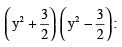Using the identity (a + b)(a – b) = a2 – b2, we have: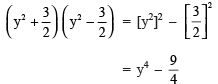( v ) (3 – 2x)(3 + 2x):
Using the identity (a + b)(a – b)
= a2 – b2,
we have: (3 – 2x)(3 + 2x)
= (3)2 – (2x)2 = 9 – 4x2

Ques 2. Evaluate the following products without multiplying directly:

(i) 103 x 107

(ii) 95 x 96

(iii) 104 x 96
Solution:
(i) We have 103 x 107
= (100 + 3)(100 + 7)
= (100)2 + (3 + 7) x 100 + (3 x 7)          [Using (x + a)(x + b) = x2 + (a + b)x + ab]
= 10000 + (10) x 100 + 21
= 10000 + 1000 + 21
= 11021
(ii) We have 95 x 96 = (100 – 5)(100 – 4)
= (100)2 + [(–5) + (–4)] x 100 + [(–5) x (–4)]           [Using (x + a)(x + b) = x2 + (a + b)x + ab]
= 10000 + [–9] x 100 + 20
= 10000 + (–900) + 20
= 9120
(iii) We have 104 x 96 = (100 + 4)(100 – 4)
= (100)2 – (4)2               [Using (a + b)(a – b) = a2 – b2]
= 10000 – 16
= 9984

Ques 3. Factorise the following using appropriate identities:

(i) 9x2 + 6xy + y2

(ii) 4y2 – 4y + 1

(iii)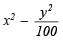Solution:
(i) We have 9x2 + 6xy + y2
= (3x)2 + 2(3x)(y) + (y)2
= (3x + y)2                     [Using a2 + 2ab + b= (a + b)2]
= (3x + y)(3x + y)
(ii) We have 4y2 – 4y + 1 = (2y)2 – 2(2y)(1) + (1)2 = (2y – 1)2 [∵ a– 2ab + b2 = (a – b)2] = (2y – 1)(2y – 1)

(iii) We have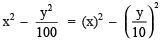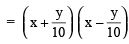[Using a2 – b2 = (a + b)(a – b)]

Ques 4. Expand each of the following, using suitable identities:
(i) (x + 2y + 4z)2

(ii) (2x – y + z)2

(iii) (–2x + 3y + 2z)2

(iv) (3a – 7b – c)2

( v ) (–2x + 5y – 3z)2

(vi)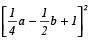Solution: (i) (x + 2y + 4z)2
We have (x + y + z)2
= x+ y+ z2 + 2xy + 2yz + 2zx
∴ (x + 2y + 4z)2
= (x)2 + (2y)2 + (4z)2 + 2(x)(2y) + 2(2y)(4z) + 2(4z)(x)
= x2 + 4y+ 16z+ 4xy + 16yz + 8zx

(ii) (2x – y + z)2
Using (x + y + z)2 = x2 + y2 + z+ 2xy + 2yz + 2zx,
we have (2x – y + z)2
= (2x)2 + (–y)2 + (z)+ 2(2x)(–y) + 2(–y)(z) + 2(z)(2x)
= 4x2 + y2 + z2 – 4xy – 2yz + 4zx

(iii) (–2x + 3y + 2z)2
Using (x + y + z)2 = x2 + y2 + z2 + 2xy + 2yz + 2zx,
we have (–2x + 3y + 2z)2
= (–2x)2 + (3y)2 + (2z)2 + 2(–2x)(3y) + 2(3y)(2z) + 2(2z)(–2x)
= 4x2 + 9y2 + 4z2 – 12xy + 12yz – 8zx

(iv) (3a – 7b – c)2
Using (x + y + z)2 = x2 + y2 + z2 + 2xy + 2yz + 2zx,
we have (3a – 7b – c)2
= (3a)2 + (–7b)2 + (–c)+ 2(3a)(–7b) + 2(–7b)(–c) + 2(–c)(3a)
= 9a+ 49b2 + c2 + (–42ab) + (14bc) + (–6ca)
= 9a2 + 49b2 + c2 – 42ab + 14bc – 6ca

(v) (–2x + 5y – 3z)2 Using (x + y + z)2 =x2 + y2 + z2 + 2xy + 2yz + 2zx,
we have (–2x + 5y – 3z)2
= (–2x)2 + (5y)2 + (–3z)2 + 2(–2x)(5y) + 2(5y)(–3z) + 2(–3z) (–2x)
=4x2 + 25y2 + 9z2 + [–20xy] + [–30yz] + [12zx]
=4x2 + 25y+ 9z2 – 20xy – 30yz + 12zx

(vi)Using (x + y + z)2 = x2 + y2 + z2 + 2xy + 2yz + 2zx, we have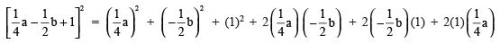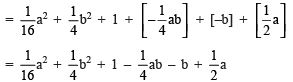Ques 5. Factorise:

(i) 4x2 + 9y+ 16z2 + 12xy – 24yz – 16xz
(ii) 2x2 + y+ 8z– 2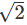xy + 4 2 yz – 8xz
Solution:
(i)4x2 + 9y2 + 16z2 + 12xy – 24yz – 16xz
= (2x)2 + (3y)2 + (–4z)2 + 2(2x)(3y) + 2(3y)(–4z) + 2(–4z)(2x)
= (2x + 3y – 4z)2                          [Using Identity V]
= (2x + 3y – 4z)(2x + 3y – 4z)

(ii) 2x2 + y2 + 8z2 – 2xy + 4yz – 8xz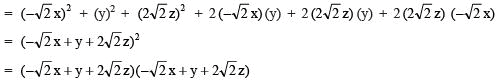Ques 6. Write the following cubes in expanded form:

(i) (2x + 1)

(ii) (2a – 3b)3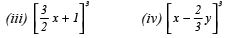Solution: Using Identity VI and Identity VII, we have

(x + y)= x+ y3 + 3xy (x + y), and

(x – y)= x3 – y3 – 3xy (x – y).

(i) (2x + 1)= (2x)+ (1)3 + 3(2x)(1)[(2x) + (1)]
= 8x3 + 1 + 6x[2x + 1]                       [Using Identity VI]
= 8x+ 1 + 12x2 + 6x
= 8x3 + 12x2 + 6x + 1

(ii) (2a – 3b)3 = (2a)3 – (3b)– 3(2a)(3b)[(2a) – (3b)]
= 8a3 – 27b– 18ab (2a – 3b)                      [Using Identity VII]
= 8a3 – 27b– [36a2b – 54ab2]
= 8a3 – 27b3 – 36a2b + 54ab2

(iii)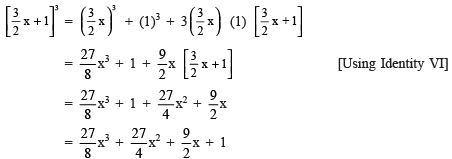(iv)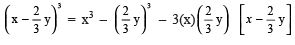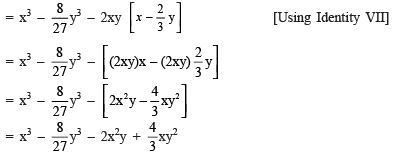Ques 7. Evaluate the following using suitable identities:

(i) (99)

(ii) (102)3

(iii) (998)3
Solution:
(i) (99)3
We have 99 = 100 – 1
∴ 993 = (100 – 1)3
= 1003 – 13 – 3(100)(1)(100 – 1)
= 1000000 – 1 – 300(100 – 1)
= 1000000 – 1 – 30000 + 300
= 1000300 – 30001
= 970299

(ii) (102)3
We have 102 = 100 + 2
∴ (102)= (100 + 2)3
= (100)3 + (2)3 + 3(100)(2) 100 + 2
= 1000000 + 8 + 600[100 + 2]
= 1000000 + 8 + 60000 + 1200
= 1061208

(iii) (998)3
We have 998 = 1000 – 2
∴ (998)3 = (1000 – 2)3
= (1000)– (2)3 – 3(1000)(2)[1000 – 2]
= 1000000000 – 8 – 6000[1000 – 2]
= 1000000000 – 8 – 6000000 – 12000
= 994011992

Ques 8. Factorise each of the following:

(i) 8a3 + b3 + 12a2b + 6ab2
(ii) 8a3 – b3 – 12a2b + 6ab
(iii) 27 – 125a3 – 135a + 225a2
(iv) 64a3 – 27b3 – 144a2b + 108ab2
(v)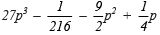Solution:
(i)8a3 + b3 + 12a2b + 6ab2 = (2a)3 + (b)3 + 6ab(2a + b)
= (2a)3 + (b)3 + 3(2a)(b)(2a + b)

= (2a + b)3                            [Using Identity VI]
= (2a + b)(2a + b)(2a + b)

(ii)8a– b3 – 12a2b + 6ab2 = (2a)3 – (b)3 – 3(2a)(b)(2a – b)
= (2a – b)3                           [Using Identity VII]
= (2a – b)(2a – b)(2a – b)

(iii) 27 – 125a3 – 135a + 225a2 = (3)– (5a)– 3(3)(5a)[3 – 5a]
= (3 – 5a)3                           [Using Identity VII]
= (3 – 5a)(3 – 5a)(3 – 5a)

(iv) 64a3 – 27b3 – 144 a2b + 108 ab2 = (4a)– (3b)3 – 3(4a)(3b)[4a – 3b]
= (4a – 3b)                          [Using Identity VII]
= (4a – 3b)(4a – 3b)(4a – 3b)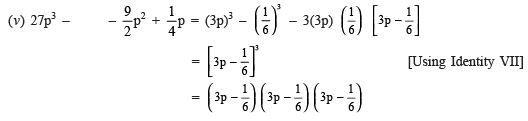Ques 9. Verify:

(i) x3 + y3 = (x + y)(x– xy + y2)
(ii) x– y= (x – y)(x2 + xy + y2)
Solution:
(i) R.H.S. = (x + y)(x– xy + y2)
= x(x2 – xy + y2) + y(x2 – xy + y2)
= x3 – x2y + xy2 + x2y – xy2 + y3
= x3 + y3 = L.H.S.

(ii) R.H.S. = (x – y)(x2 + xy + y2)
= x(x2 + xy + y2) – y(x2 + xy + y2)
= x+ x2y + xy– x2y – xy2 – y3
= x– y= L.H.S.

Ques 10. Factorise each of the following: (i) 27y+ 125z3 (ii) 64m3 – 343n3

REMEMBER
I. x3 + y3 = (x + y)(x2 + y2 – xy) II. x– y3 = (x – y)(x2 + y+ xy)

Solution: (i) Using the identity (x3 + y3) = (x + y)(x2 – xy + y2),
we have 27y3 + 125z3 = (3y)+ (5z)3
= (3y + 5z)    [(3y)2 – (3y)(5z) + (5z)2]
= (3y + 5z)(9y2 – 15yz + 25z2)

(ii) Using the identity x3 – y3 = (x – y)(x+ xy + y2),
we have 64m3 – 343n
= (4m)3 – (7n)
= (4m – 7n)[(4m)2 + (4m)(7n) + (7n)2]
= (4m – 7n)(16m2 + 28mn + 49n2)

Ques 11. Factorise 27x+ y+ z3 – 9xyz.
Solution:

REMEMBER
x3 + y3 + z3 – 3xyz = (x + y + z)(x+ y2 + z2 – xy – yz – zx)

We have 27x+ y+ z3 – 9xyz = (3x)3 + (y)+ (z)3 – 3(3x)(y)(z)
∴ Using the identity x+ y3 + z– 3xyz = (x + y + z)(x+ y2 + z2 – xy – yz – zx),
we have  (3x)3 + (y)3 + (z)– 3(3x)(y)(z)
= (3x + y + z)[(3x)2 + y2 + z2 – (3x x y) – (y x z) – (z x 3x)]
= (3x + y + z)(9x2 + y2 + z2 – 3xy – yz – 3zx)
Ques 12. Verify that x3 + y3 + z3 – 3xyz =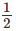(x + y + z)[(x – y)2 + (y – z)2 + (z – x)2]
Solution:
R.H.S. =(x + y + z)[(x – y)2 + (y – z)2 + (z – x)2]
=(x + y + z)[(x2 + y2 – 2xy) + (y2 + z2 –2yz) + (z2 + x2 – 2xz)]
=(x + y + z)[x2 + y2 + y2 + z2 + z2 + x2 – 2xy – 2yz – 2zx]
=(x + y + z)[2(x2 + y2 + z2 – xy – yz – zx)]
= 2 xx (x + y + z)(x2 + y2 + z2 – xy – yz – zx)
= (x + y + z)(x2 + y2 + z2 – xy – yz – zx)
= x3 + y3 + z3 – 3xyz = L.H.S.

Ques 13. If x + y + z = 0, show that x3 + y3 + z3 = 3xyz.
Solution:
Since x + y + z = 0
∴ x + y = –z or (x + y)3
= (–z)or x3 + y3 + 3xy(x + y)
= –zor x3 + y3 + 3xy(–z)
= –z                      [∵ x + y = (–z)]
or x3 + y3 – 3xyz = –z3
or (x3 + y3 + z3) – 3xyz
= 0 or (x3 + y3 + z3) = 3xyz
Hence, if x + y + z = 0, then (x3 + y3 + z3) = 3xyz.
Ques 14. Without actually calculating the cubes, find the value of each of the following: (i) (–12)3 + (7)3 + (5)3 (ii) (28)3 + (–15)3 + (–13)3
Solution:
(i) (–12)3 + (7)+ (5)3
Let x = –12, y = 7 and z = 5
Then x + y + z = –12 + 7 + 5 = 0
We know that if x + y + z = 0, then x3 + y3 + z= 3xyz.
∴ (–12)3 + (7)3 + (5)3
= 3 [(–12)(7)(5)]                   [∵ (–12) + 7 + 5 = 0]
= 3[–420]
= –1260
Thus, (–12)3 + (7)+ (5)3 = –1260

(ii) (28)3 + (–15)3 + (–13)
Let x = 28, y = –15 and z = –13
∴ x + y + z = 28 – 15 – 13 = 0
We know that if x + y + z = 0,
then x3 + y3 + z3 = 3xyz.
∴ (28)+ (–15)3 + (–13)3
= 3(28)(–15)(–13)             [∵ 28 + (–15) + (–13) = 0]
= 3(5460)
= 16380
Thus, (28)3 + (–15)3 + (–13)3 = 16380

Ques 15. Give possible expressions for the length and breadth of each of the following rectangles, in which their areas are given: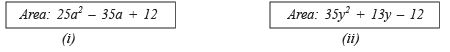REMEMBER
Area of a rectangle = (Length) x (Breadth)
Solution:
(i) Area = 25a2 – 35a + 12
We have to factorise the polynomial: 25a2 – 35a + 12
Splitting the co-efficient of a, we have –35 = (–20) + (–15) [∵ 25 x 12 = 300 and (–20) x (–15)
= 300] 25a2 – 35a + 12 = 25a2 – 20a – 15a + 12
∴= 5a(5a – 4) – 3(5a – 4) = (5a – 4)(5a – 3)
Thus, the possible length and breadth are (5a – 3) and (5a – 4).

(ii) Area = 35y+ 13y – 12
We have to factorise the polynomial 35y+ 13y – 12.

Splitting the middle term, we get 13y = 28y – 15y [∵ 28 x (–15)
= –420 and –12 x 35 = – 420]
∴ 35y2 + 13y – 12
= 35y2 + 28y – 15y – 12
= 7y(5y + 4) –3(5y + 4)
= (5y + 4)(7y – 3)
Thus, the possible length and breadth are (7y – 3) and (5y + 4).

Ques 16. What are the possible expressions for the dimensions of the cuboids whose volumes are given below?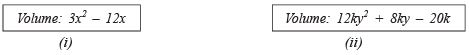REMEMBER

Volume of a cuboid = (Length) x (Breadth) x (Height) Solution:

(i) Volume = 3x2 – 12x On factorising 3x2 – 12x,
we have 3x2 – 12x = 3[x2 – 4x]
= 3 x [x(x – 4)] = 3 x x x (x – 4)

∴ The possible dimensions of the cuboid are: 3, x and (x – 4) units.

(ii) Volume = 12ky2 + 8ky – 20k
We have 12ky2 + 8ky – 20k
= 4[3ky2 + 2ky – 5k] = 4[k(3y2 + 2y – 5)]
= 4 x k x (3y2 + 2y – 5)
= 4k[3y2 – 3y + 5y – 5] (Splitting the middle term)
= 4k[3y(y – 1) + 5(y – 1)]
= 4k[(3y + 5)(y – 1)]
= 4k x (3y + 5) x (y – 1)
Thus, the possible dimensions are: 4k, (3y + 5) and (y – 1) units.

## Mathematics (Maths) Class 9

192 videos|230 docs|82 tests

,

,

,

,

,

,

,

,

,

,

,

,

,

,

,

,

,

,

,

,

,

;